Above Earth

To what height must a boy be raised above the earth in order to see one-fifth of its surface.

Result

h =  4252 km

Solution:Leave us a comment of example and its solution (i.e. if it is still somewhat unclear...):Be the first to comment!To solve this example are needed these knowledge from mathematics:

Need help calculate sum, simplify or multiply fractions? Try our fraction calculator. Pythagorean theorem is the base for the right triangle calculator.

Next similar examples:

1. Felix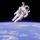Calculate how much land saw Felix Baumgartner after jump from 32 km above ground. The radius of the Earth is R = 6378 km.
2. MO SK/CZ Z9–I–3John had the ball that rolled into the pool and it swam in the water. Its highest point was 2 cm above the surface. Diameter of circle that marked the water level on the surface of the ball was 8 cm. Determine the diameter of John ball.
3. Elevation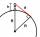What must be the elevation of an observer in order that he may be able to see an object on the earth 536 km away? Assume the earth to be a smooth sphere with radius 6378.1 km.
4. AirplaneAviator sees part of the earth's surface with an area of 200,000 square kilometers. How high he flies?
5. Tropical, mild and arctic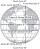How many percents of the Earth's surface lies in the tropical, mild and arctic range? The border between the ranges is the parallel 23°27 'and 66°33'.
6. PilotHow high is the airplane's pilot to see 0.001 of Earth's surface?
7. Spherical cap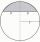What is the surface area of a spherical cap, the base diameter 20 m, height 2 m.
8. Spherical cap 4What is the surface area of a spherical cap, the base diameter 20 m, height 2.5 m? Calculate using formula.
9. Spherical capFrom the sphere of radius 18 was truncated spherical cap. Its height is 12. What part of the volume is spherical cap from whole sphere?
10. Spherical tank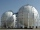The tank of a water tower is a sphere of radius 35ft. If the tank is filled to one quarter of full, what is the height of the water?A domed stadium is in the shape of spherical segment with a base radius of 150 m. The dome must contain a volume of 3500000 m³. Determine the height of the dome at its centre to the nearest tenth of a meter.Calculate the area of a spherical cap, which is part of an area with base radius ρ = 9 cm and a height v = 3.1 cm.Spherical segment with height h=6 has a volume V=134. Calculate the radius of the sphere of which is cut this segment.The vessel hemispherical hollow is filled with water to a height of 10 cm =. How many liters of water are inside if the inside diameter of the hollow is d = 28cm?Place a part of the sphere on a 4.6 cm cylinder so that the surface of this section is 20 cm2. Determine the radius r of the sphere from which the spherical cap was cut.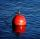A 0.5 meter spherical float is used as a location mark for a fishing boat anchor. It floats in salt water. Find the depth to which the float sinks if the material of which the float is made weighs 8 kilograms per cubic meter and salt water weighs 1027 kg/m